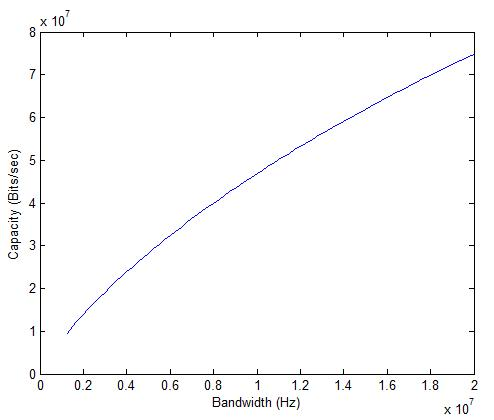# Shannon Capacity of LTE (Effective)

In the previous post we calculated the Shannon Capacity of LTE as a function of bandwidth. We now calculate the capacity as a function of SNR (bandwidth fixed at 20MHz and signal power varied). We also use the concept of effective bandwidth to get a more realistic estimate of the capacity. The modified Shannon Capacity formula is given as:

C=B_eff*log2(1+SNR)

where

B_eff=Bandwidth*eff1*eff2*eff3*eff4

eff1=0.9=due to adjacent channel leakage ratio and practical filter issues

eff2=0.93=due to cyclic prefix

eff3=0.94=due to pilot assisted channel estimation

B_eff=0.57*B

Therefore

C=0.57*B*log2(1+SNR)

Note: This is the capacity in a SISO channel with no fading.

# Shannon Capacity of LTE (Ideal)

Shannon Capacity of LTE in AWGN can be calculated by using the Shannon Capacity formula:

C=B*log2(1+SNR)

or

C=B*log2(1+P/(B*No))

The signal power P is set at -90dBm, the Noise Power Spectral Density No is set at 4.04e-21 W/Hz (-174dBm/Hz) and the bandwidth is varied from 1.25MHz to 20MHz.It is seen that the capacity increases from about 10Mbps to above 70Mbps as the bandwidth is varied from 1.25MHz to 20MHz (keeping the signal power constant). It must be noted that this is the capacity with a single transmit and single receive antenna (MIMO capacity would obviously be higher).

# Shannon Capacity of a GSM Channel in Fading Environment

In the previous post we calculated the Shannon Capacity of a 200kHz GSM channel in AWGN (Additive White Gaussian Noise). However, in a practical scenario the capacity is limited by time varying fading and interference. Let us consider a fading channel with four possible states corresponding to SNRs of 15dB, 10dB, 5dB and 0dB. The probability of these states is 0.50, 0.25, 0.15 and 0.10 respectively. The Shannon Capacity of such a channel is given as (assuming that the channel state information is known at the receiver):

C=Σ B*log2(1+SNRi)* p(SNRi)

C=B*(Σ log2(1+SNRi)* p(SNRi))

C=(200e3)*(log2(1+31.62)*0.50+log2(1+10.00)*0.25+log2(1+3.16)*0.15+log2(1+1)*0.10)

C=757.43kbps

Assuming that only one out of eight time slots is allocated to any user the Shannon Capacity of a GSM channel is reduced to 94.68kbps.

Note: The contribution of the high SNR states dominates the capacity of the channel. The contribution of the four states in terms of percentage capacity is given as 66.38%, 22.84%, 8.14% and 2.64%.

# Shannon Capacity of a GSM Channel

We know that GSM bit rates can vary from a few kbps to a theoretical maximum of 171.2kbps (GPRS). But what is the actual capacity of a 200kHz GSM channel. We can use the Shannon Capacity Theorem to find this capacity.

C=B*log2(1+SNR)

or

C=B*log2(1+P/N)

The noise power can be found by using the following formula:

N=B*No=k*T*B=(1.38e-23)*(293)*(200e3)=8.08e-16W=-121dBm

Let us now assume a signal power 0f -90dBm. This gives us an SNR of 31dB or 1258.9 on linear scale. The capacity can thus be calculated as:

C=200e3*log2(1+1258.9)=2.06Mbps

This is the capacity if all time slots are allocated to a single user. If only one time slot is allocated to a user the capacity would be reduced to 257.48kbps.

# CDMA vs FDMA Capacity (Mbps)

We saw previously that the channel capacity of a WCDMA system is severely limited by the Multiple Access Interference (MAI). Now let us consider the case where the 5MHz channel is divided equally among 20 users such that each user has a bandwidth of 250kHz. Keeping the transmit power for the narrow band signal the same as the wideband signal the signal to noise ratio would improve tremendously (since there is no MAI and the noise power is reduced by a factor of 20). Thus each narrowband channel would have a capacity of 2.99Mbps giving a combined capacity of 59.83Mbps for the 5MHz channel. The main drawback of an FDMA system is that the capacity of each narrowband channel is fixed at 2.99Mbps. However the capacity of a WCDMA user is dependent upon the number of users varying from 38Mbps for a single user to 5Mbps for two users (10Mbps combined) to 370kbps for 20 users (7.4Mbps combined). The higher capacity of FDMA when combined with dynamic allocation of channels builds the case for OFDMA based techniques.

Note: For the narrowband case the signal power is -80dBm and noise power is -116dBm resulting in an SNR of 36dB.